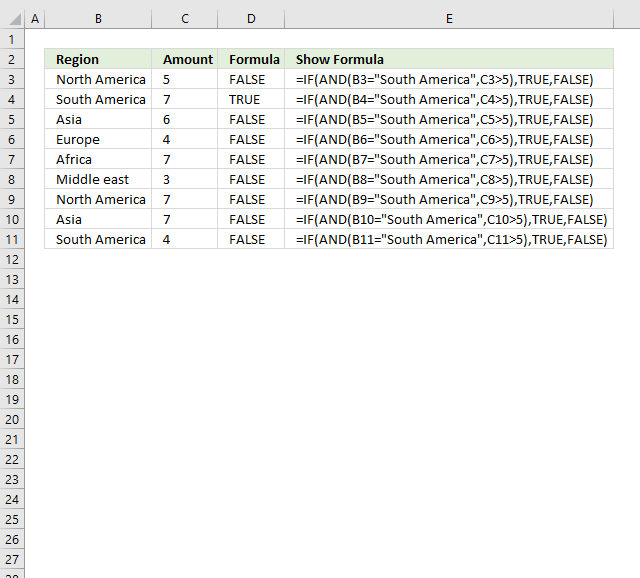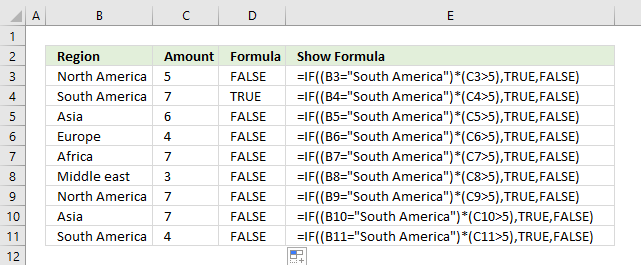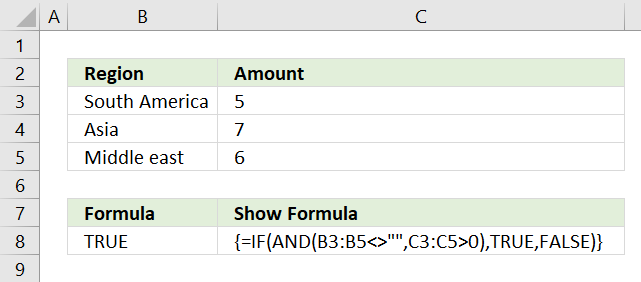Author: Oscar Cronquist Article last updated on April 02, 2018The AND function allows you to have multiple conditions in an IF function, you can have up to 254 arguments. An argument is an input value given to a function. You construct a logical expression that you use as an argument in the AND function.

The IF function above checks two conditions, the "Region" value must match a text string and the "Amount" value must be larger than a number. If both conditions return TRUE the AND function returns TRUE.

IF REGION = value AND Amount > number then TRUE Else FALSE

In other words, all logical tests in each argument in the AND function must return TRUE for the AND function to return TRUE. The AND function returns FALSE if at least one argument returns FALSE.You can shorten the formula somewhat by enclosing each logical expression with parentheses and then multiply the conditions. The asterisk * means multiply.

The first logical expression in cell B4 is (B4="South America") and it returns TRUE, the second expression (C4>5) also returns TRUE. TRUE multiplied with TRUE is 1.

TRUE is the same thing as 1 and FALSE is 0 (zero). If you multiply boolean values the outcome is always 0 (zero) or 1.The array formula above lets you check if all values in cell range B3;B5 are not empty and if all numbers in C3:C5 are above 0 (zero). It returns TRUE if all conditions are TRUE.

There are many conditions in above formula and the array formula lets you do this without problems.

To enter an array formula press and hold CTRL + SHIFT simultaneously, then press Enter once. Release all keys.

The formula bar now shows the formula enclosed with curly brackets telling you that you entered the formula successfully. Don't enter the curly brackets yourself.